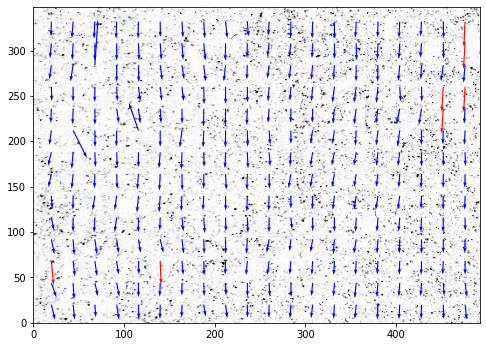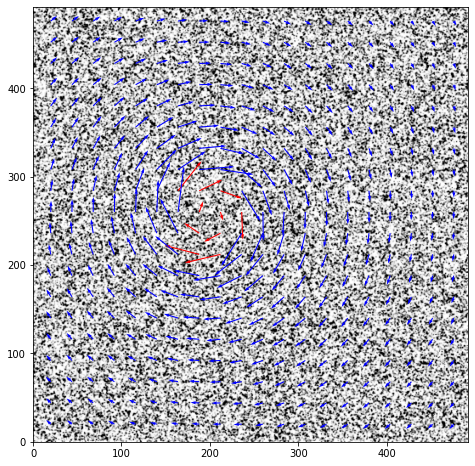OpenPIV tutorial of all test cases¶

In :
from openpiv import tools, pyprocess, scaling, validation, filters
import numpy as np
import glob
import matplotlib.pyplot as plt
%matplotlib inline
In :
%watermark -v -m -p numpy,openpiv -g -b
Python implementation: CPython
Python version       : 3.9.6
IPython version      : 7.26.0

numpy  : 1.21.2
openpiv: 0.23.6

Compiler    : MSC v.1916 64 bit (AMD64)
OS          : Windows
Release     : 10
Machine     : AMD64
Processor   : Intel64 Family 6 Model 58 Stepping 9, GenuineIntel
CPU cores   : 8
Architecture: 64bit

Git branch: master

In :
# set of typical parameters
window_size = 32 # pixels 32 x 32 pixels interrogation window, in frame A.
overlap = 16 # overlap is 8 pixels, i.e. 25% of the window
search_size = 40  # pixels 64 x 64 in frame B, avoids some peak locking for
# large displacements
In :
def openpiv_default_run(im1, im2, window_size, overlap, search_size):
""" default settings for OpenPIV analysis using
extended_search_area_piv algorithm for two images

Inputs:
im1,im2 : str,str = path of two image
"""

u, v, sig2noise = pyprocess.extended_search_area_piv(frame_a.astype(np.int32),
frame_b.astype(np.int32),
window_size=window_size,
overlap=overlap,
dt=1,
search_area_size=search_size,
sig2noise_method='peak2mean',
correlation_method='circular',
normalized_correlation=True)
x, y = pyprocess.get_rect_coordinates(frame_a.shape,
search_size,
overlap)
# 5% lowest range
u, v, mask = validation.sig2noise_val(u, v,
sig2noise,
threshold = np.percentile(sig2noise,2.5))
u, v = filters.replace_outliers( u, v, method='localmean',
max_iter=3, kernel_size=3)
x, y, u, v = scaling.uniform(x, y, u, v, scaling_factor = 1. )

x, y, u, v = tools.transform_coordinates(x, y, u, v)

tools.save(x, y, u, v, mask, list_of_images+'.txt' )
fig,ax = plt.subplots(figsize=(8,8))

tools.display_vector_field(list_of_images+'.txt',
on_img=True,image_name=list_of_images,
scaling_factor=1.,
ax=ax)
#     tools.display_vector_field(list_of_images+'.txt',
#                                         scaling_factor=1.,
#                                         ax=ax)
In :
alist_filter = ['jpg','bmp','png','tif','tiff']

# all test cases in /openpiv/examples/
list_of_tests = glob.glob('../test*')
list_of_tests.sort()

list_of_images = []
for test in list_of_tests:

list_of_files = glob.glob(test+'/*.*')
list_of_files.sort()
list_of_images = [f for f in list_of_files if f[-3:] in alist_filter]
if len(list_of_images) > 0:
openpiv_default_run(list_of_images,list_of_images,window_size, overlap, search_size)